# How to Demodulate an AM Waveform

## Chapter 5 - Radio Frequency Demodulation

Learn about two circuits that can extract the original information from an amplitude-modulated carrier signal.

At this point we know that modulation refers to intentionally modifying a sinusoid such that it can carry lower-frequency information from a transmitter to a receiver. We also have covered many details related to the different methods—amplitude, frequency, phase, analog, digital—of encoding information in a carrier wave.

But there is no reason to integrate data into a transmitted signal if we cannot extract that data from the received signal, and this is why we need to study demodulation. Demodulation circuitry ranges from something as simple as a modified peak detector to something as complex as coherent quadrature downconversion combined with sophisticated decoding algorithms performed by a digital signal processor.

### Creating the Signal

We’ll use LTspice to study techniques for demodulating an AM waveform. But before we demodulate we need something that is modulated.

In the AM modulation page, we saw that four things are needed to generate an AM waveform. First, we need a baseband waveform and a carrier waveform. Then we need a circuit that can add an appropriate DC offset to the baseband signal. And finally, we need a multiplier, since the mathematical relationship corresponding to amplitude modulation is multiplying the shifted baseband signal by the carrier.

The following LTspice circuit will generate an AM waveform.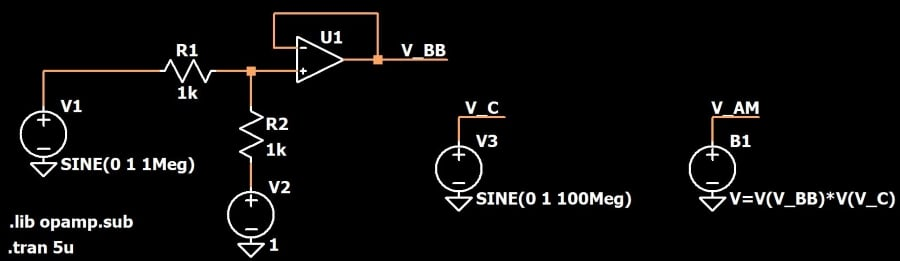• V1 is a 1 MHz sine wave voltage source that provides the original baseband signal.
• V3 produces a 100 MHz sine wave for the carrier.
• The op-amp circuit is a level shifter (it also reduces the input amplitude by half). The signal coming from V1 is a sine wave that swings from –1 V to +1 V, and the output of the op-amp is a sine wave that swings from 0 V to +1 V.
• B1 is an “arbitrary behavioral voltage source.” Its “value” field is a formula rather than a constant; in this case the formula is the shifted baseband signal multiplied by the carrier waveform. In this way B1 can be used to perform amplitude modulation.

Here is the shifted baseband signal: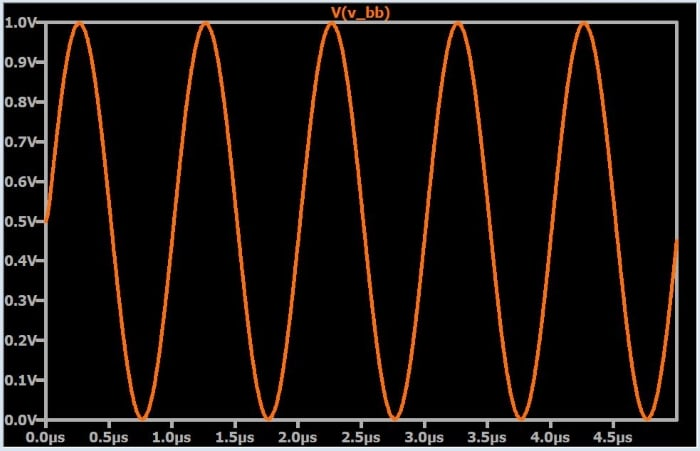And here you can see how the AM variations correspond to the baseband signal (i.e., the orange trace that is mostly obscured by the blue waveform):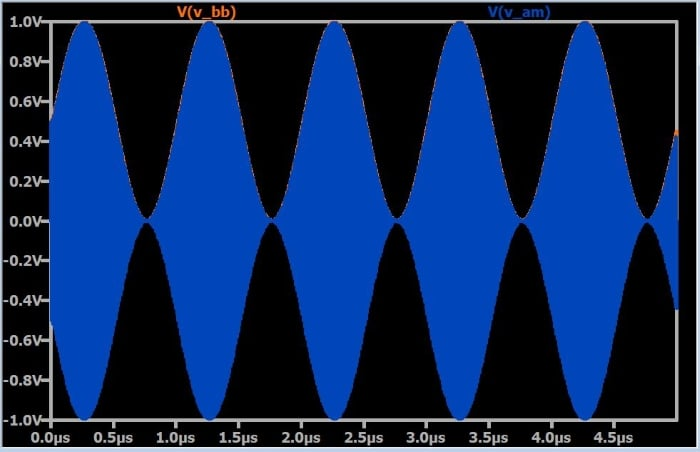Zooming in reveals the individual cycles of the 100 MHz carrier frequency.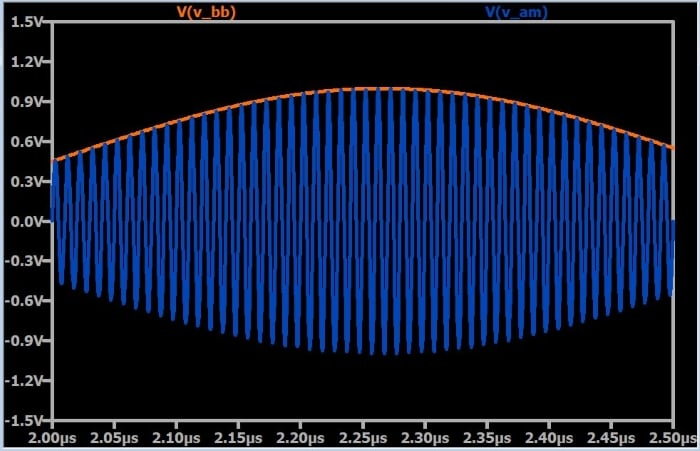### Demodulation

As discussed in the AM modulation page, the multiplication operation used to perform amplitude modulation has the effect of transferring the baseband spectrum to a band surrounding the positive carrier frequency (+fC) and the negative carrier frequency (–fC). Thus, we can think of amplitude modulation as shifting the original spectrum upward by fC and downward by fC. It follows, then, that multiplying the modulated signal by the carrier frequency will transfer the spectrum back to its original position—i.e., it will shift the spectrum downward by fC such that it is once again centered around 0 Hz.

#### Option 1: Multiplication and Filtering

The following LTspice schematic includes a demodulating arbitrary behavioral voltage source; B2 multiplies the AM signal by the carrier.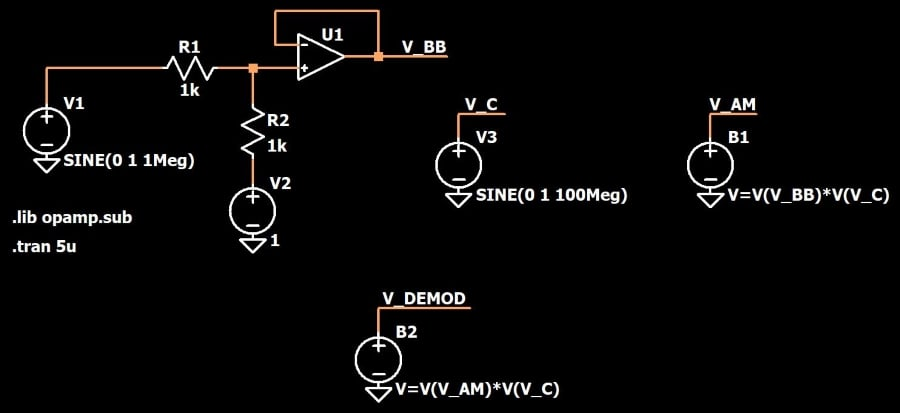And here is the result: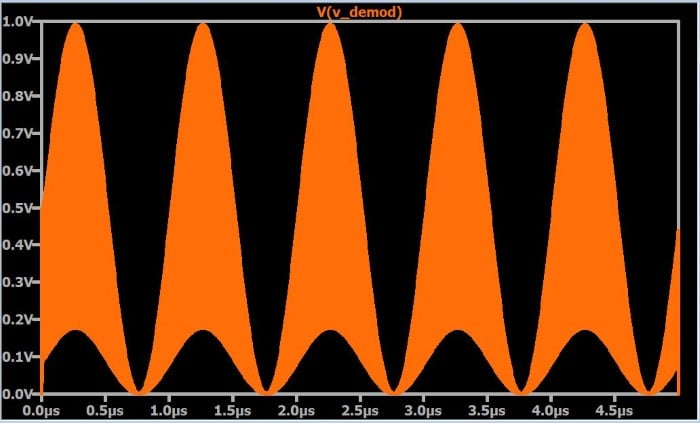This definitely does not look correct. If we zoom in, we see the following: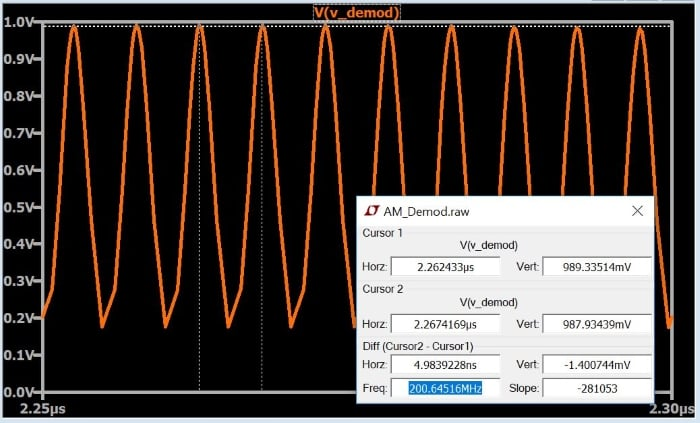And this reveals the problem. After amplitude modulation, the baseband spectrum is centered around +fC. Multiplying the AM waveform by the carrier shifts the baseband spectrum down to 0 Hz, but it also shifts it up to 2fC (in this case 200 MHz), because (as stated above) multiplication moves the existing spectrum up by fC and down by fC.

It is clear, then, that multiplication alone is not sufficient for proper demodulation. What we need is multiplication and a low-pass filter; the filter suppresses the spectrum that was shifted up to 2fC. The following schematic includes an RC low-pass filter with a cutoff frequency of ~1.5 MHz.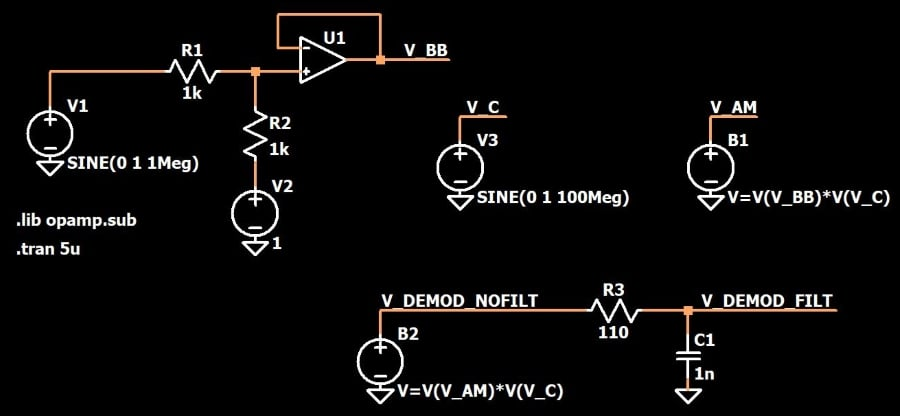And here is the demodulated signal: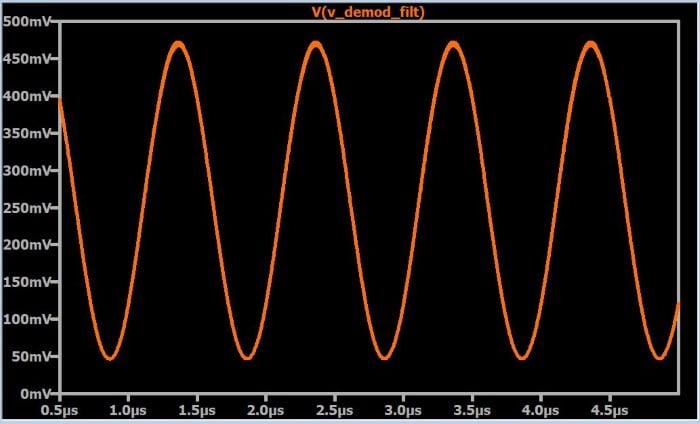This technique is actually more complicated than it appears because the phase of the receiver’s carrier-frequency waveform must be synchronized with the phase of the transmitter’s carrier. This is discussed further in page 5 of this chapter (Understanding Quadrature Demodulation).

#### Option 2: Peak Detector

As you can see above in the plot that shows the AM waveform (in blue) and the shifted baseband waveform (in orange), the positive portion of the AM “envelope” matches the baseband signal. The term “envelope” refers to the carrier’s variations in sinusoidal amplitude (as opposed to the variations in the instantaneous value of the waveform itself). If we could somehow extract the positive portion of the AM envelope, we could reproduce the baseband signal without using a multiplier.

It turns out that it is quite easy to convert the positive envelope into a normal signal. We start with a peak detector, which is just a diode followed by a capacitor. The diode conducts when the input signal is at least ~0.7 V above the voltage on the capacitor, and otherwise it acts like an open circuit. Thus, the capacitor maintains the peak voltage: if the current input voltage is lower than the capacitor voltage, the capacitor voltage doesn’t decrease because the reverse-biased diode prevents discharge.

However, we don’t want a peak detector that will retain the peak voltage for a long period of time. Instead, we want a circuit that retains the peak relative to the high-frequency variations of the carrier waveform, but does not retain the peak relative to the lower-frequency variations of the envelope. In other words, we want a peak detector that holds the peak only for a short period of time. We accomplish this by adding parallel resistance that allows the capacitor to discharge. (This type of circuit is called a “leaky peak detector,” where “leaky” refers to the discharge path provided by the resistor.) The resistance is chosen such that the discharge is slow enough to smooth out the carrier frequency and fast enough to not smooth out the envelope frequency.

Here is an example of a leaky peak detector for AM demodulation: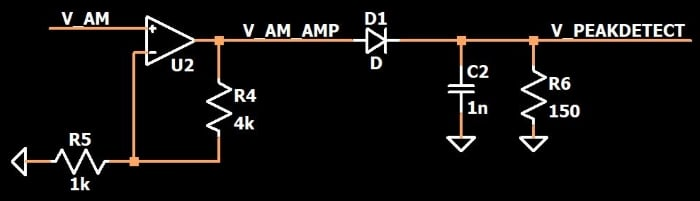Note that I have amplified the AM signal by a factor of five in order to make the peak detector’s input signal larger relative to the diode’s forward voltage. The following plot conveys the general result that we are trying to achieve with the leaky peak detector.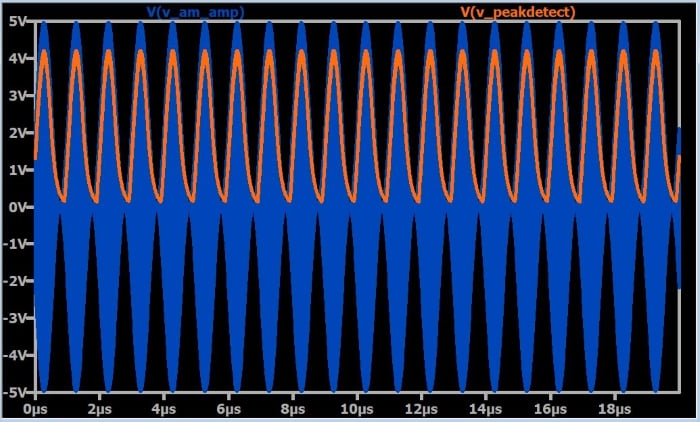The final signal exhibits the expected charge/discharge characteristic: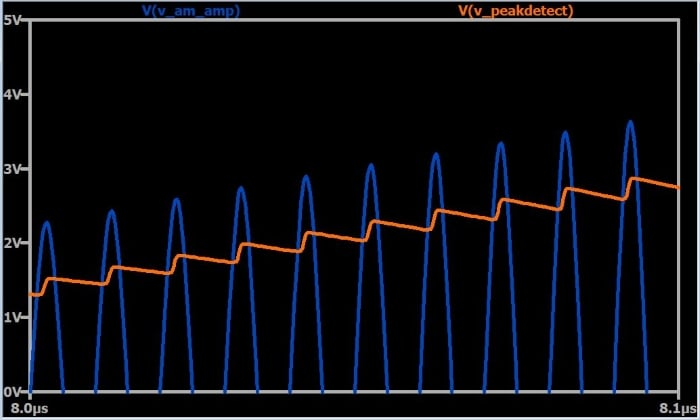A low-pass filter could be used to smooth out these variations.

### Summary

• In LTspice, an arbitrary behavioral voltage source can be used to create an AM waveform.
• AM waveforms can be demodulated using a multiplier followed by a low-pass filter.
• A simpler (and lower-cost) approach is to use a leaky peak detector, i.e., a peak detector with parallel resistance that allows the capacitor to discharge at an appropriate rate.
• Share
Published under the terms and conditions of the Design Science License